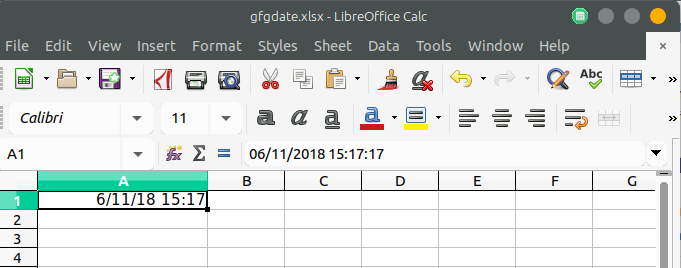PHP | Spreadsheet | Setting a date and/or time value in a cell

• Difficulty Level : Easy
• Last Updated : 19 Nov, 2018

In PHP Spreadsheet, the date or time values are stored in an excel sheet in the form of timestamp which is a floating point value. So in order to store date/time in a human-readable format, need to calculate the correct excel timestamp and finally set a number format mask.

Example:

 getActiveSheet();    // Set the number format mask so that the excel timestamp // will be displayed as a human-readable date/time\$spreadsheet->getActiveSheet()->getStyle('A1')    ->getNumberFormat()    ->setFormatCode(    \PhpOffice\PhpSpreadsheet\Style\NumberFormat::FORMAT_DATE_DATETIME    );   // Get current date and timestamp// Convert to an Excel date/time\$dateTime = time(); \$excelDateValue = \PhpOffice\PhpSpreadsheet\Shared\Date::PHPToExcel(                  \$dateTime );    // Set cell A1 with the Formatted date/time value\$sheet->setCellValue('A1',\$excelDateValue);   // Write an .xlsx file \$writer = new Xlsx(\$spreadsheet);  // Save .xlsx file to the current directory\$writer->save('gfgdate.xlsx');?>

Output:My Personal Notes arrow_drop_up